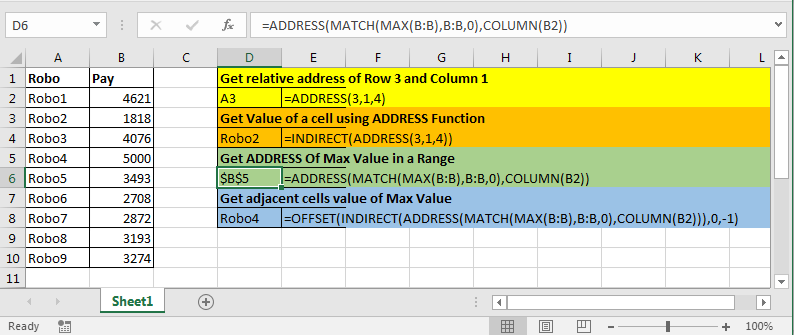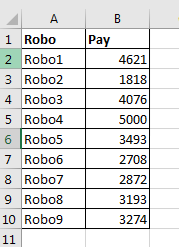# The ADDRESS Function in Excel

The ADDRESS function simply returns the address of a cell by using row number and column number.Row_num: The Row number

Col_num: The Column number

[abs_num]: Optional Argument.  It has four possible inputs, 1, 2, 3, and 4. If omitted or 1, returned address will be absolute eg \$A\$1. 2 returns absolute row and relative column eg A\$1.3 returns relative row and absolute column \$A1. 4 returns relative address eg A1.

[a1]: Optional Argument. Has two possible inputs, 0 and 1. If omitted, it will return A1-style . 0 returns output in RC style.

[sheet_name]: Optional Argument. If sheet name is provided, add it with address along ! mark. Eg. mysheet!A1.

IMPORTANT: The address returned is in text format. It's not an actual reference.
Now let's have some examples:1. Get relative address of Row 3 and Column 1.

The above ADDRESS formula will return A3.

1. Get Value of a cell using ADDRESS Function

The above formula will return value at \$A\$3

1. Get ADDRESS of Max Value in a Range.

We have this table.Write this formula anywhere to get the address of Max Value.

MAX function finds max value in range B:B
MATCH looks for that MAX value in B:B range and returns index or say row number.
COLUMN returns column number of B2.
Now, ADDRESS has row_num and col_num. And this all it needs.

1. Get adjacent cells value of Max Value

Many times you would require to get the name of a person getting max or min value. One approach is this.

Since the address returned by ADDRESS function is text, we use INDIRECT so that OFFSET function takes it as a real address.
we move 1 column left to max cell using -1. And finally, offset returns name of Robo that is getting max pay.

So, I explained the ADDRESS function here and gave some examples in which ADDRESS is most useful. But as we know everyone’s need is different, let me know your need in the comments section below. We will get it together.

Related Articles:

How To Look Up Address in Excel

Excel ROW function

Excel COLUMN Function in Excel

Relative and Absolute Reference in Excel

Expanding References in Excel

Absolute reference in Excel

Popular Articles:

50 Excel Shortcuts to Increase Your Productivity

How to use the VLOOKUP Function in Excel

How to use the COUNTIF function in Excel 2016

How to Use SUMIF Function in Excel

Terms and Conditions of use

The applications/code on this site are distributed as is and without warranties or liability. In no event shall the owner of the copyrights, or the authors of the applications/code be liable for any loss of profit, any problems or any damage resulting from the use or evaluation of the applications/code.